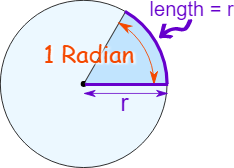# Calculating angles

I have been wondering for a long while now how angles are calculated for rotation. They typically use the CFrame of one part and the other part and perform an operation on them. I cannot wrap my head around this. I have asked a similar question before but “angles are angles” is not quite a helpful response.

5 LikesHope this helped!

6 Likes

So first, a solid definition for a `CFrame`:

A `CFrame` is something that describes a combined position and angle.

That is literally all it is. All CFrame constructors revolve around creating CFrames with specific properties. e.g. `CFrame.new's` 3-number constructor creates a CFrame with a position, but no angle, while `CFrame.Angles`, `CFrame.fromOrientation`, etc., creates a CFrame specifically with an angle.

You are probably talking about inverse CFrames here, which I can explain.
So an inverse CFrame is a CFrame that is the reciprocal of another CFrame. If you multiply a CFrame by its Inverse, it will make all the position and angle differences cancel out and spit out an identity CFrame.
The best way to imagine the inverse CFrame is to imagine that you are offsetting the center of the world by your part’s CFrame.

Let’s say you have a part that is 10 studs above the center:

``````part.CFrame = CFrame.new(0, 10, 0)
``````

If you have a point that is 1 stud above the part, it would just be 11 studs above center:

``````point = CFrame.new(0, 11, 0)
``````

If you were to multiply this point by the inverse of the part’s CFrame, it would give you the point’s CFrame as if the part was the new center:

``````relativeCFrame = part.CFrame:Inverse() * point
print(relativeCFrame.Position) --> 0, 1, 0
``````

Similarly, if you were to change the angle of the CFrame, that would also affect the inverse.

So say you had another part’s CFrame that was at the center, but turned 90 degrees so it was facing in the X direction:

``````part2.CFrame = CFrame.Angles(0, math.rad(-90), 0)
print(part2.CFrame.LookVector) --> almost 1, 0, 0
``````

If you were to create a point that was facing the front of the part, say, 5 studs ahead, it would just be moved 5 studs in the X direction:

``````point = CFrame.new(5, 0, 0)
``````

By that logic, if `part2` became the new center, then the `point's` relative position would be five studs forward from the center in the Z direction:

``````relativeCFrame = part2.CFrame:Inverse() * point
print(relativeCFrame.Position) --> almost 0, 0, 5
``````

So, if you have two part CFrames, you can multiply the inverse of one part’s CFrame by the other to get a relative CFrame between the two:

``````relativeCFrame = part.CFrame:Inverse() * part2.CFrame
``````

You can use this `relativeCFrame` to apply a constant relative position from one part to the other, if you so wanted:

``````-- in a loop:
part.CFrame = -- whatever you want
part2.CFrame = part.CFrame * relativeCFrame
``````

e.g:

The code I used:

``````local relativeCFrame = part.CFrame:Inverse() * part2.CFrame

local i = 0
while true do
local dt = wait()
i = (i + dt/3)%1 -- loops between [0, 1) in 3 seconds

-- changes the position using sine curves

-- changes the angle

-- gives `part2` a position relative to `part`
part2.CFrame = part.CFrame * relativeCFrame
end
``````

You can pseudo-decompose the `relativeCFrame` into its original components, and you’ll notice two of the CFrames cancel out, leaving the original CFrame from `part2`:

``````part2.CFrame = part.CFrame * relativeCFrame

-- decomposed: [   these two would cancel out!   ]
part2.CFrame = part.CFrame * part.CFrame:Inverse() * part2.CFrame
``````

However, these don’t truly cancel out because the `part's` CFrame changed after the relative CFrame was created, which is what creates the static relative offset from `part` without keeping `part2` in the same position.

Also, a weird thing, I tried switching around the two terms for `part2's` assignment CFrame:

``````-- from:
part2.CFrame = part.CFrame * relativeCFrame
-- to
part2.CFrame = relativeCFrame * part.CFrame
``````

This caused `part2` to stay in an offsetted position from `part`, but the position wasn’t really relative:

It makes sense though, since positions then angles are applied to the prior CFrame, and you are basically taking a static position and offsetting by the position then the angle.

Hopefully, that helps with understanding inverse CFrames better. Have a great day24 Likes

Nice thorough and very readable tutorial as a response! This could be on Community Tutorials.

2 Likes